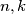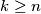### IMO Shortlist 1999 problem N5

Kvaliteta:
Avg: 0,0
Težina:
Avg: 8,0
Let$n,k$ be positive integers such that n is not divisible by 3 and$k \geq n$. Prove that there exists a positive integer$m$ which is divisible by$n$ and the sum of its digits in decimal representation is$k$.
Izvor: Međunarodna matematička olimpijada, shortlist 1999